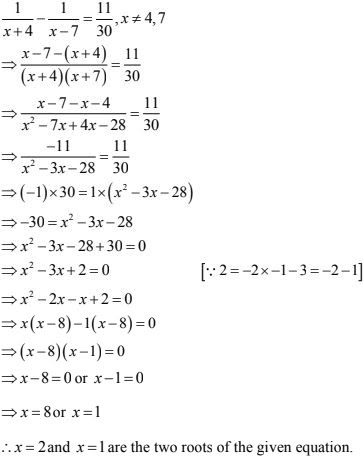# RD Sharma Solutions Chapter 8 Quadratic Equations Exercise 8.3 Class 10 Maths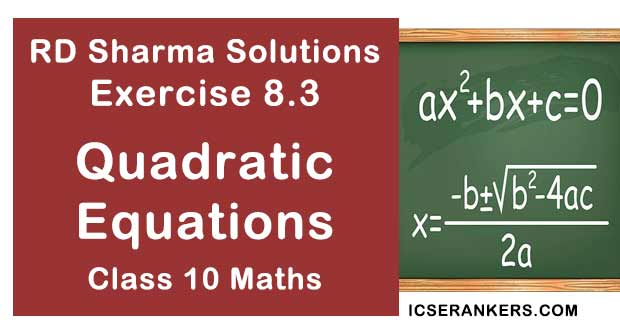Chapter Name RD Sharma Chapter 8 Quadratic Equations Book Name RD Sharma Mathematics for Class 10 Other Exercises Exercise 8.1Exercise 8.2Exercise 8.4Exercise 8.5Exercise 8.6 Related Study NCERT Solutions for Class 10 Maths

### Exercise 8.3 Solutions

Solve the following quadratic equations by factorization:

1. (x - 4)(x + 2) = 0

Solution

We have
(x - 4)(x + 2) = 0
⇒ either (x - 4) = 0 or (x + 2) = 0
⇒ x = 4 or x = -2
Thus, x = 4 and x = -2 are two roots of the equation(x - 4)(x + 2) = 0

2. (2x + 3)(3x - 7) = 0

Solution

We have,
(2x + 3)(3x- 7) = 0
⇒ (2x + 3) = 0 or (3x- 7) = 0
⇒ 2x = - 3 or 3x = 7
⇒ x = -3/2 or x = 7/3
Thus, x = -3/2 and x = 7/3 are two roots of the equation(2x + 3)(3x - 7) = 0

3. 4x2 + 5x = 0

Solution

We have 4x2 + 5x = 0
⇒ x (4x + 5) = 0
⇒ either x = 0 or 4x + 5 = 0
⇒ x = 0 or 4x = -5
⇒ x = 0 or x = -5/4
Thus, x = 0 and x = -5/4 are two roots of equation 4x2 + 5x = 0

4. 9x2 - 3x - 2 = 0

Solution

We have  9x2 - 3x - 2 = 0
⇒  9x2 - 6x + 3x - 2 = 0
⇒ 3x (3x - 2) + 1(3x - 2) = 0
⇒ (3x - 2)(3x + 1) = 0
⇒ either 3x - 2 = 0 or 3x + 1 = 0
⇒ either 3x - 2 = 0 or 3x + 1 = 0
⇒ 3x = 2 or 3x = - 1
⇒ x = 2/3 or x = -1/3
Thus, x = 2/3 and x = -1/3 are two roots of the equation 9x2 - 3x - 2 = 0

5. 6x2 - x - 2 = 0

Solution

We have 6x2 - x - 2 = 0
⇒ 6x2 + 3x - 4x - 2 = 0
⇒ 3x(2x + 1) - 2(2x + 1) = 0
⇒ (2x + 1)(3x - 2) = 0
⇒ either 2x + 1 = 0 or 3x - 2 = 0
⇒ 2x = - 1 or 3x = 2
⇒ x = -1/2 or x = 2/3
Thus, x = -1/2 and x = 2/3 are two roots of the equation 6x2 - x - 2 = 0

6. 6x2 + 11x + 3 = 0

Solution

We have
6x2 + 11x + 3 = 0
⇒ 6x2 + 9x + 2x + 3 = 0
⇒ 3x(2x + 3) + 1 (2x + 3) = 0
⇒ (2x + 3)(3x + 1) = 0
⇒ 2x + 3 = 0 or x = -1/3
Thus, x = -3/2 and x = -1/3 are the two roots of the given equation.

7. 5x2 - 3x - 2 = 0

Solution

We have
5x2 - 3x - 2 = 0
⇒ 5x2 - 5x + 2x - 2 = 0
⇒ 5x(x - 1) + 2(x - 1) = 0
⇒ (x - 1)(5x + 2) = 0
⇒ (x - 1) = 0 or 5x + 2 = 0
⇒ x = 1 or x = -2/5
∴ x = 1 and x = -2/5 are the two roots of the given equation.

8.  48x2 - 13x - 1 = 0

Solution

We have
48x2 - 13x - 1 = 0
⇒ 48x2 - 16x + 3x - 1 = 0
⇒ 16x (3x - 1) + 1(3x - 1) = 0
⇒ (3x - 1)(16x + 1) = 0
⇒ 3x - 1 = 0 or 16x + 1 = 0
⇒ x = 1/3 or x = -1/16
∴ x= -1/16 and x = 1/3 are the two roots of the given equation.

9. 3x2 = - 11x - 10

Solution

We have
3x2 = - 11x - 10
⇒ 3x2 + 11x + 10 = 0
⇒ 3x2 + 6x + 5x + 10 = 0
⇒ 3x(x + 2) + 5(x + 2) = 0
⇒ (x + 2)(3x + 5) = 0
⇒ (x + 2) = 0 or x = -5/3
∴ x = -2 and x = -5/3  are the two roots at the quadratic equation 3x2 = -11x - 10

10. 25x(x + 1) = - 4

Solution

We have
(x + 1) = -4
⇒(25x) × x + (25x) × 10 - 4
⇒ 25x2 + 25x + 4 = 0  [25×4 = 100 ⇒ 25 = 20 + 5 ⇒ 100 = 20×5]
⇒ 25x2 + 20x + 5x + 4 = 0
⇒ 5x(5x + 4) + 1(5x + 4) = 0
⇒ (5x + 4)(5x + 1) = 0
⇒ 5x + 4 = 0 or 5x + 1 = 0
⇒ x = -4/3 or x = -1/3
∴ x = -4/3 and x = -1/5 are the two solutions of the quadratic equation 25x(x + 1) = -4

11. 10x - 1/x = 3

Solution

We have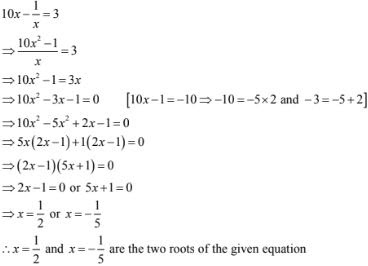12. 2/22 - 5/x + 2 = 0

Solution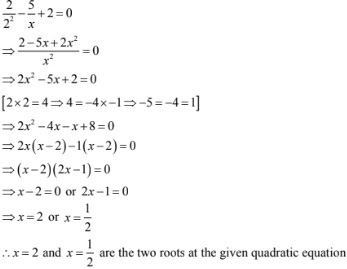13. 4√3x2 + 5x - 2√3 = 0
Solution
We have,
4√3x2 + 5x - 2√3 = 0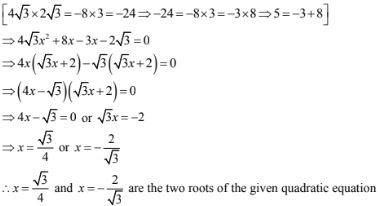14. √2x2 - 3x - 2√2 = 0
Solution
We have,15. a2x2 - 30bx + 2b2 = 0
Solution
We have,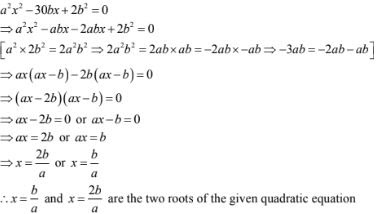16. x2 - (√2 + 1)x + √2 = 0
Solution
We have,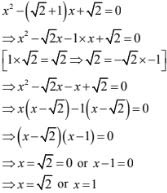∴ x = 1 and x = √2 are the roots of the given quadratic equation

17. x2 - (√3 + 1)x + √3 = 0
Solution
x2 - (√3 + 1)x + √3 = 0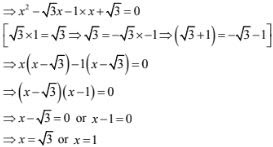18. 4x2 + 4bx - (a2 - b2) = 0
Solution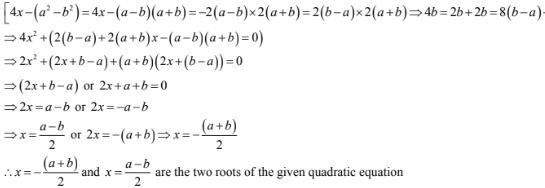19. ax2 + (4a2 - 3b)x - 12ab = 0
Solution
We have,
ax2 + (4a2 - 3b)x - 12ab = 0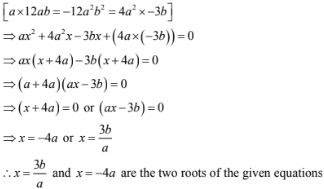20. (x - 1/2)2  = 4
Solution
We have,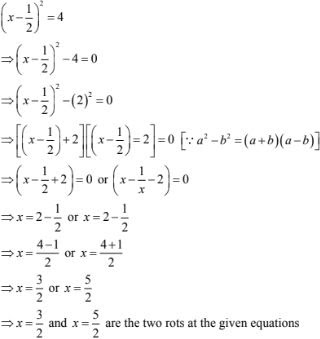21. x2 - 4√2 x + 6 = 0
Solution
We have,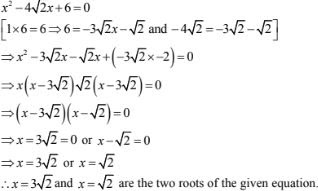22. (x + 3)/(x + 2) = (3x - 7)/(2x - 3)
Solution⇒ (x + 3)(2x - 3) = (x + 2)(3x - 7)
⇒ 2x2 - 3x + 6x - 9 = 3x2 - x - 14
⇒ 2x2 + 3x - 9 = 3x2 - x - 14
⇒ x2 - 3x - x - 14 + 9 = 0
⇒ x2 - 4x - 5 = 0
[1x - 5 = -5 - 4 = -5 + 1]
⇒ x2 - 5x + x - 5 = 0
⇒ x(x - 5) + 1(x - 5) = 0
⇒ (x - 5)(x + 1) = 0
⇒ x - 5 = 0 or x + 1 = 0
⇒ x = 5 or x = - 1
∴ x = 5 and x = - 1 are the two roots of the given quadratic equation.

23 2x/(x - 4) + (2x -5)/(x- 3) = 25/3
Solution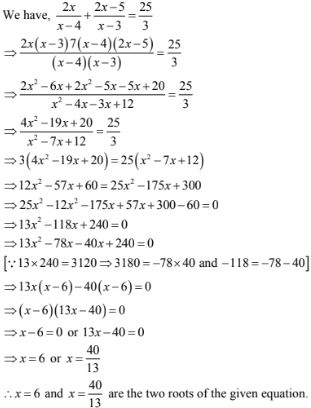24. (x + 3)/(x - 2) - (1 - x)/x = 17/4
Solution
We have,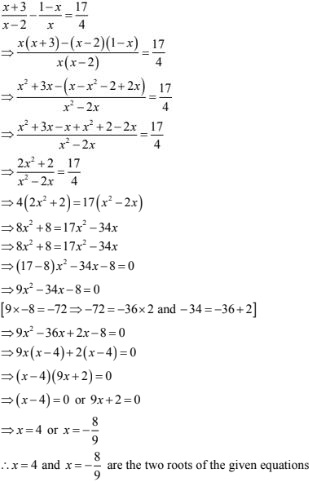25. (x - 3)/(x + 3) - (x + 3)/(x - 3) = 48/7, x ≠ 3, x ≠ - 3
Solution
- 4, 9/4

26. 1/(x - 2) + 2/(x - 1) = 6/x, x ≠ 0
Solution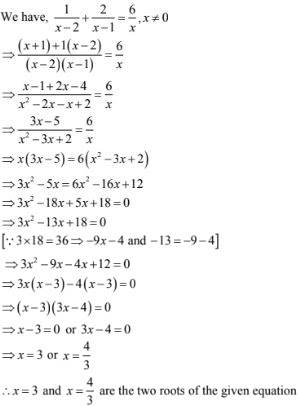27. (x + 1)/(x - 1) - (x - 1)/(x + 1) = 5/6, x ≠ 1 and x ± - 1
Solution
We have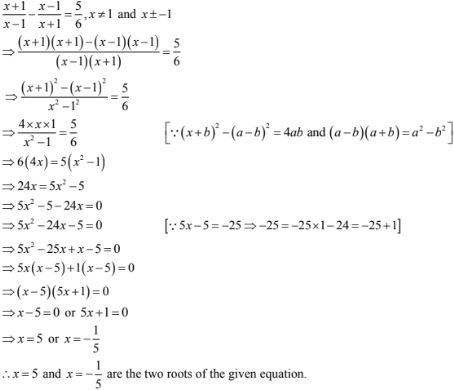28. (x - 1)/(2x + 1) - (2x + 1)/(x - 1) = 5/2, x ≠ -1/2, 1
Solution
We have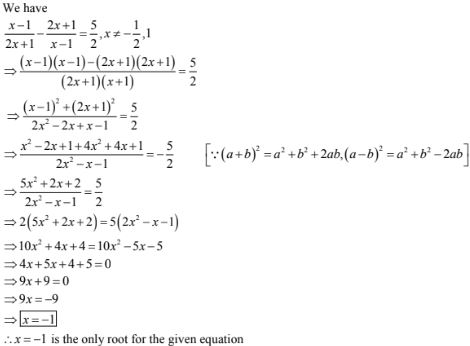29. 3x2 - 14x - 5 = 0
Solution30. (m/n)x2 + n/m = 1 - 2x
Solution
We have given,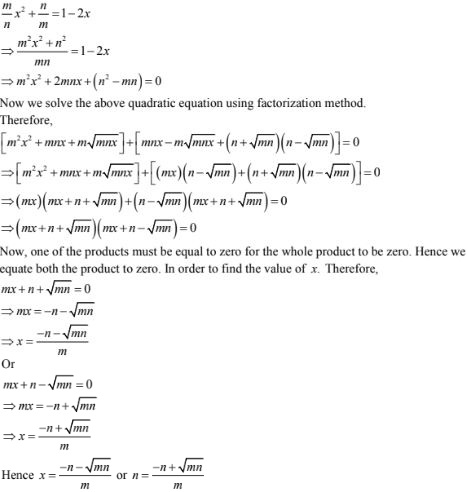31. (x - a)/(x - b) + (x - b)/(x - a) = a/b + b/a
Solution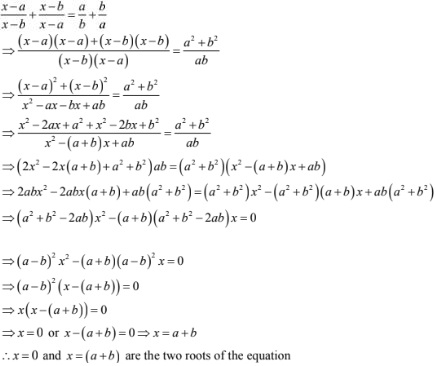32. 1/(x -1)(x - 2) + 1/(x - 2)(x - 3) + 1/(x - 3)(x - 4) = 1/6
Solution
We have,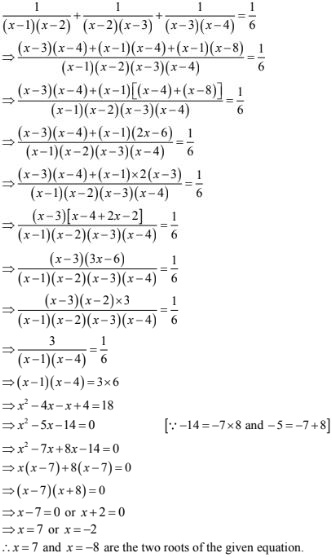33. (x - 5)(x - 6) = 25/(24)2
Solution
We have,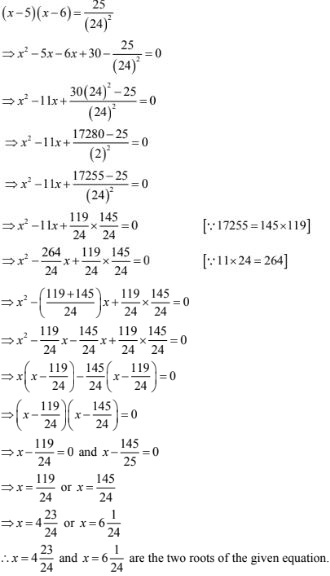34. 7x + 3/x  = 35 + 3/5
Solution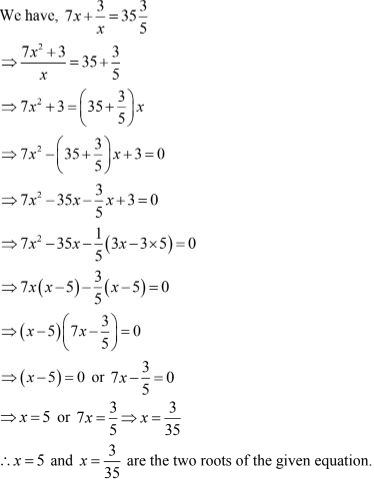35. a/(x - a) + b/(x - b) = 2c/(x - c)
Solution
We have,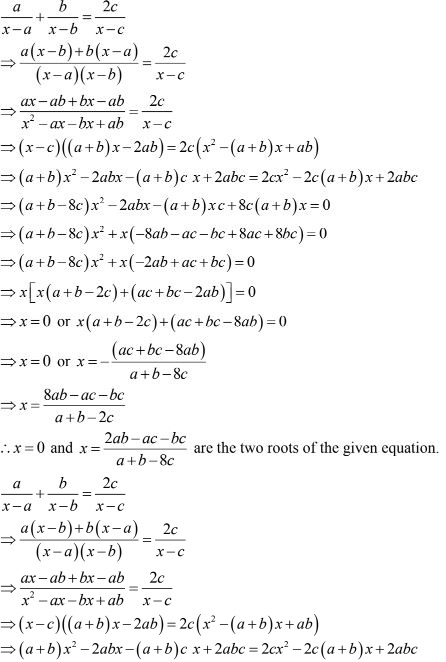36. x2 + 2ab = (2a + b)x
Solution
We have
x2  + 2ab = (2a + b)x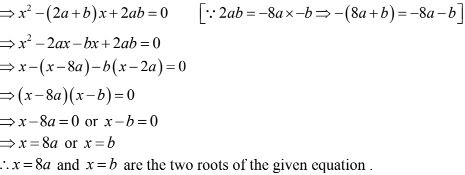37. (a + b)2 x2 -(4ab)x - (a - b)2  = 0
Solution
We have ,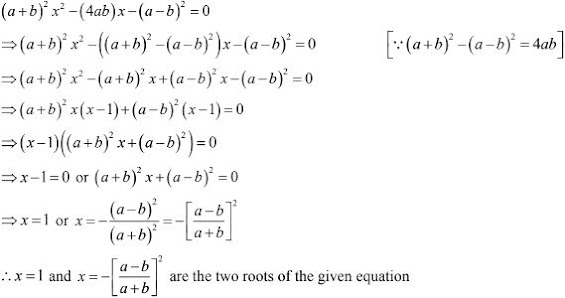38. a(x2 + 1) - x(a2 + 1) = 0
Solution
We have
a(x2 + 1) - x(a2 + 1) = 0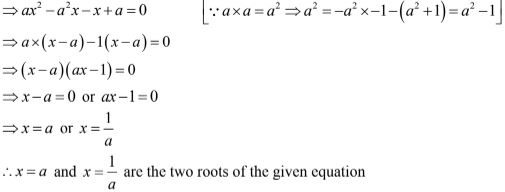39. x2 - x - x(a + 1) = 0
Solution
We have,
x2 - x - x(a + 1) = 0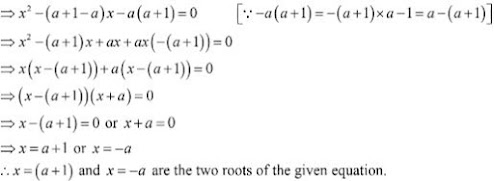40. x2 + (a + 1/a)x + 1 = 0
Solution
We have,
x2  + (a + 1/a)a + 1 = 0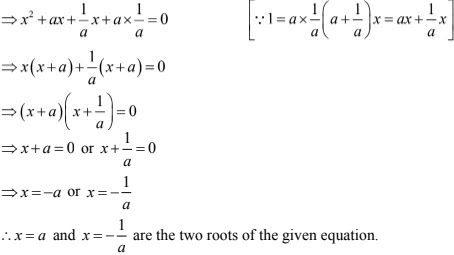41. abx2 + (b2 - ac)x - bc = 0
Solution
We have,
abx2 + (b2 - ac)x - bc = 0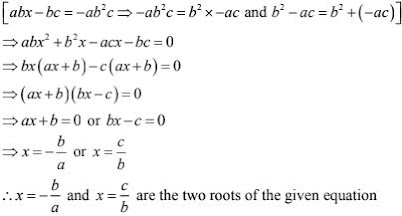42. a2b2x2 + b2x - a2x - 1 = 0
Solution
We have,
a2b2x2 + b2x - a2x - 1 = 0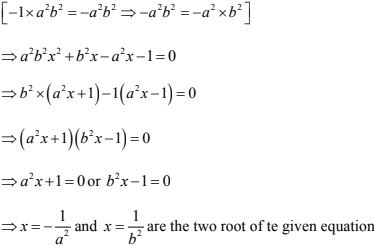43. (x - 1)/(x - 2) + (x - 3)/(x - 4) =  3 + 1/3, x ≠ 2 and x ≠ 4
Solution
We have,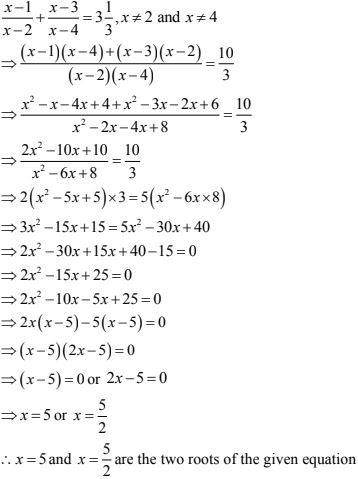44. 3x2 - 2√6x + 2 = 0
Solution
We have 3x2 - 2√6x + 2 = 0
Now we solve the above quadratic equation using factorization method.
Therefore45. 1/(x - 1) - 1/(x + 5) = 6/7, x ≠ 1, - 5
Solution
We have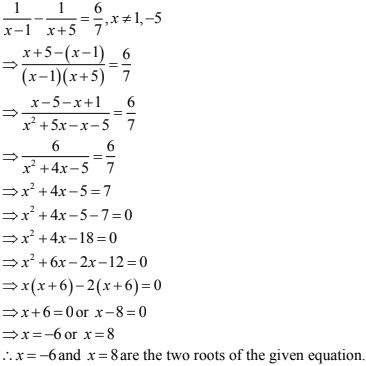46. 1/x - 1/(x - 2) = 3, x ≠ 0, 2
Solution
We have,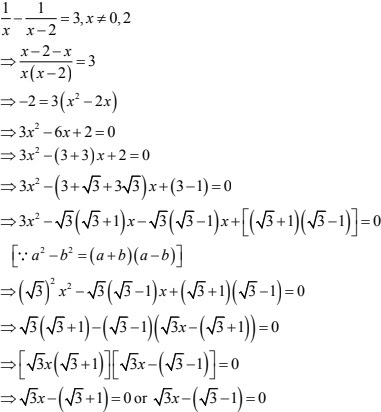47. x - 1/x = 3, x ≠ 0
Solution
We have,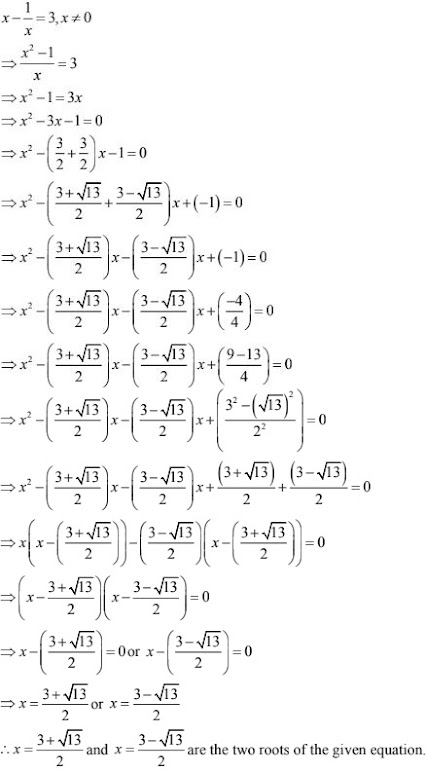48. 1/(x + 4) - 1/(x- 7) = 11/30, x ≠ 4, 7
Solution
We have,